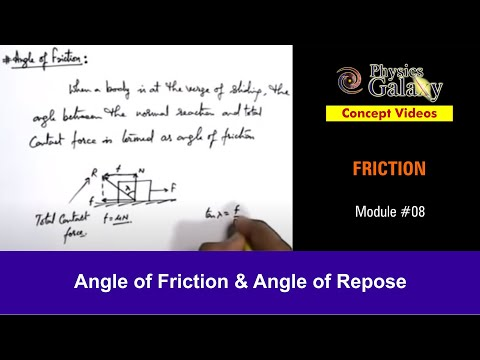### Main Difference

Both the terms Angle of Friction and Angle of Repose are widely used in fields like statics and dynamics and most prominently in the field of mechanical engineering. Both the terms have interference of friction in it and that is why angle of friction is also referred as the angle of repose. Though one knowing their definitions and usage under different conditions can easily differentiate it between both the terms. Angle of Friction is the angle between the normal force (N) and the resultant force (R) of normal force and friction, whereas Angle of Repose is the angle of maximum slope, where an object placed just begins to slide. In other words we can say that it is that maximum angle where a body or object can be tilted from the horizontal without falling or collapsing.

### Comparison Chart

 Angle of Friction Angle of Repose Definition Angle of Friction is the angle between the normal force (N) and the resultant force (R) of normal force and friction. Angle of Repose is the angle of maximum slope, where an object placed just begins to slide. Applicable Angle of friction is applicable between the rigid surfaces. Angle of repose is used for incline surfaces, especially the granular materials in soil mechanics. Angle Hypothetical Angle Real Angle

### What is Angle of Friction?

Angle of Friction is the angle between the normal force (N) and the resultant force (R) of normal force and friction. Actually it is the hypothetical angle between the resultant force and the horizon. The Angle of Friction is attained when the failure happens in response to the shearing stress. When we talk about the friction, here we generally talk about the dry friction which is further subdivided into static friction and kinetic friction. To determine the value of angle of friction an experiment is conducted. The equation F = µ R explains the angle of friction as the static friction creating hindrance in movement of an object reaches the upper limit, µ is the coefficient of friction in the case and R is the normal force.

### Angle of Repose?

It is quite similar to the angle of friction as it has interference of friction and is about the ability of making object stand firm at the maximum angle without collapsing or falling. Actually it is the angle from where on wards object might start sliding horizontally. We can define Angle of Repose as the angle of maximum slope, where an object placed just begins to slide. Angle of Repose is about the granular materials and other such materials as it is mainly used in soil mechanics. Sediment stones, sand, and snow are the few of the granular materials used in soil mechanics that are placed at angles where object avoids sliding down. In our daily lives, we can see Antlions using the angle of repose concept as they make the pots in sand to catch their prey. They set an angle where the other insect slid downward and they catch them.

### Angle of Friction vs. Angle of Repose

• Angle of friction is the angle between the normal force (N) and the resultant force (R) of normal force and friction, whereas Angle of Repose is the angle of maximum slope, where an object placed just begins to slide.
• Angle of friction is applicable between the rigid surfaces, whereas angle of repose is used for incline surfaces, especially the granular materials in soil mechanics.
• Angle of repose has the real angle while angle of friction is based on the hypothetical angle between the resultant force and the horizon.